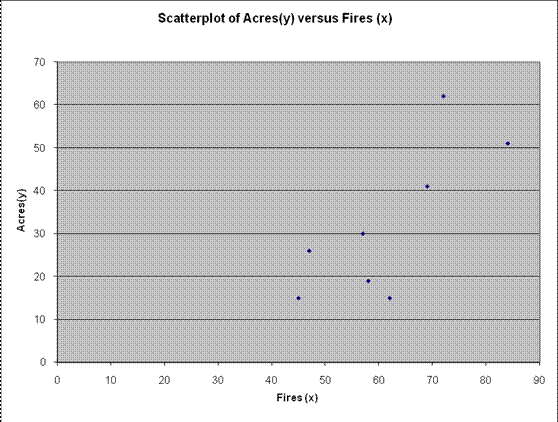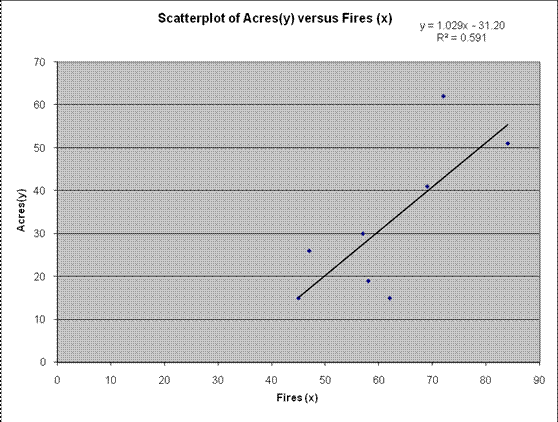# Linear Regression Tutorial

## Why is linear regression important?

Linear Regression is among the most used models in Statistics. The main reasons are its simplicity and often time strong predictive power.

Also, the calculation of the regression equation given sample data for two variables is very simple from an algebraic point of view, and it comes available in basic spreadsheets apps like Excel and in most scientific calculators.

. See below a list of relevant sample problems, with step by step solutions.

## Sample Linear Regression problems: Regression Equation Calculations

Question 1: Linear Regression Formula The formulas for the least square line were found by solving the system of equations

$nb+m\left( \sum{x} \right)=\sum{y}$

$b\left( \sum{x} \right)+m\left( \sum{x^2} \right)=\sum{xy}$

How do you calculate linear regression by hand? Solve these equations for b and m to show that

\begin{align} & m=\frac{n\left( \sum{xy} \right)-\left( \sum{x} \right)\left( \sum{y} \right)}{n\left(\sum{{{x}^{2}}} \right)-{{\left( \sum{x} \right)}^{2}}} \\ & b=\frac{\sum{y-m\left( \sum{x}\right)}}{n} \\ \end{align}

Solution: From

$nb+m\left( \sum{x} \right)=\sum{y}$

$b\left( \sum{x} \right)+m\left( \sum{x^2} \right)=\sum{xy}$

we have two equations and two unknowns (m and b)

We get that multiplying the first equation by $$\left( \sum{x} \right)$$ and the second by -n we get

\begin{align} & nb\left( \sum{x} \right)+m{{\left( \sum{x} \right)}^{2}}=\left( \sum{y}\right)\left(\sum{x} \right) \\ & -nb\left( \sum{x} \right)-mn\left( {{\sum{x}}^{2}}\right)=n\sum{xy} \\ \end{align}

$m\left( {{\left( \sum{x} \right)}^{2}}-n\left( {{\sum{x}}^{2}} \right) \right)=\left( \sum{x} \right)\left(\sum{y} \right)-n\left( \sum{xy} \right)$

$\Rightarrow \,\,\,\,m=\frac{\left( \sum{x} \right)\left( \sum{y} \right)-n\left( \sum{xy} \right)}{{{\left( \sum{x} \right)}^{2}}-n\left( {{\sum{x}}^{2}} \right)}=\frac{n\left( \sum{xy} \right)-\left( \sum{x} \right)\left( \sum{y} \right)}{n\left( {{\sum{x}}^{2}} \right)-{{\left( \sum{x} \right)}^{2}}}$

Now, from this equation:

$nb+m\left( \sum{x} \right)=\left( \sum{y} \right)$

we can solve for b :

$nb+m\left( \sum{x} \right)=\left( \sum{y} \right)\,\,\Rightarrow \,\,\,nb=\left( \sum{y} \right)-m\left( \sum{x} \right)\,\Rightarrow \,\,\,b=\frac{\left( \sum{y} \right)-m\left( \sum{x} \right)}{n}$

Question 2: Determine the correlation coefficient and do a graph of the regression line with the regression coefficient for the following set of data.

Forest fires and acres burned. The number of fires and the number of acres burned are as follows

 Fires (x) 72 69 58 47 84 62 57 45 Acres(y) 62 41 19 26 51 15 30 15

Solution: (a) The following scatterplot is obtained:Based on the scatterplot above, we observe that there is a moderate to strong degree of positive linear association.

(b) On the other hand, we have the following table shows the calculations needed in order to compute Pearson correlation: We get

 X Y X² Y² X·Y 72 62 5184 3844 4464 69 41 4761 1681 2829 58 19 3364 361 1102 47 26 2209 676 1222 84 51 7056 2601 4284 62 15 3844 225 930 57 30 3249 900 1710 45 15 2025 225 675 Sum 494 259 31692 10513 17216

The Pearson correlation r is computed as

$r = \frac{n\sum\limits_{i=1}^{n}{{{x}_{i}}{{y}_{i}}}-\left( \sum\limits_{i=1}^{n}{{{x}_{i}}} \right)\left( \sum\limits_{i=1}^{n}{{{y}_{i}}} \right)}{\sqrt{n\left( \sum\limits_{i=1}^{n}{x_{i}^{2}} \right)-{{\left( \sum\limits_{i=1}^{n}{{{x}_{i}}} \right)}^{2}}}\sqrt{n\left( \sum\limits_{i=1}^{n}{y_{i}^{2}} \right)-{{\left( \sum\limits_{i=1}^{n}{{{y}_{i}}} \right)}^{2}}}} = \frac{8 \times {17216}-{494}\times {259}}{\sqrt{8\times {31692}-{494}^{2}}\sqrt{8\times 10513-{259}^{2}}}$

$=0.7692$

(c) The coefficient of determination is

${{r}^{2}}={0.7692}^{2}= {0.5917}$

which means that 59.17% of the variation in Acres(y) is explained by Fires (x) .

(d) We need to calculate the regression equation. The regression coefficients are computed

$b=\frac{n\left( \sum\limits_{i=1}^{n}{{{x}_{i}}{{y}_{i}}} \right)-\left( \sum\limits_{i=1}^{n}{{{x}_{i}}} \right)\left( \sum\limits_{i=1}^{n}{{{y}_{i}}} \right)}{n\left( \sum\limits_{i=1}^{n}{x_{i}^{2}} \right)-{{\left( \sum\limits_{i=1}^{n}{{{x}_{i}}} \right)}^{2}}}=\frac{8 \times {17216}-{494}\times {259}}{8 \times {31692}-{494}^{2}}= 1.0297$

and

$a=\bar{y}-b \bar{x}={32.375}{+} {1.0297}\,\cdot \, {61.75} = {-31.208}$

This means that the regression equation is

$\hat{y}= {-31.208}{+}{1.0297}\,x$

Graphically:Question 3: You have conducted a study to determine if the average time spent in the computer lab each week and the course grade in a computer course were correlated. Using the data given below, what conclusion would you draw on this issue?

 student # hours in lab Course Grade 1 20 96 2 11 51 3 16 62 4 13 58 5 89 6 15 81 7 10 46 8 10 51

Solution: The following table shows the calculations needed in order to compute Pearson correlation r : We get

 X Y X² Y² X·Y 20 96 400 9216 1920 11 51 121 2601 561 16 62 256 3844 992 13 58 169 3364 754 17 89 289 7921 1513 15 81 225 6561 1215 10 46 100 2116 460 10 51 100 2601 510 Sum 112 534 1660 38224 7925

The Pearson correlation r is computed as

$r = \frac{n\sum\limits_{i=1}^{n}{{{x}_{i}}{{y}_{i}}}-\left( \sum\limits_{i=1}^{n}{{{x}_{i}}} \right)\left( \sum\limits_{i=1}^{n}{{{y}_{i}}} \right)}{\sqrt{n\left( \sum\limits_{i=1}^{n}{x_{i}^{2}} \right)-{{\left( \sum\limits_{i=1}^{n}{{{x}_{i}}} \right)}^{2}}}\sqrt{n\left( \sum\limits_{i=1}^{n}{y_{i}^{2}} \right)-{{\left( \sum\limits_{i=1}^{n}{{{y}_{i}}} \right)}^{2}}}} = \frac{8 \times {7925}-{112}\times {534}}{\sqrt{8\times {1660}-{112}^{2}}\sqrt{8\times 38224-{534}^{2}}}$

$=0.9217$

We want to test for the significance of the correlation coefficient. More specifically, we want to test

\begin{align}{{H}_{0}}:\rho {=} 0 \\ {{H}_{A}}:\rho {\ne} 0 \\ \end{align}

In order to test the null hypothesis, we use a t-test. The t-statistics is computed as

$t= r \sqrt{\frac{n-2}{1-{{r}^{2}}}}= {0.9217} \times \sqrt{\frac{6}{1-{0.9217}^2}}= {5.8198}$

The two-tailed p-value for this test is computed as

$p=\Pr \left( |{{t}_{6}}|>5.8198 \right)=0.0011$

Since $$p = 0.0011 {<} 0.05$$ , and this means that we reject the null hypothesis H 0 .

Hence, we have enough evidence to support the claim that the correlation between Number of hours in lab and Course Grade is significantly different from zero.

Since there is sufficient evidence to assume that there is a meaningful linear association, you can now use this regression equation calculator to estimate its regression coefficients, or you can calculate the regression coefficients by hand using the formula presented above.